# Resources tagged with: Graphs

Filter by: Content type:
Age range:
Challenge level:

### There are 45 results

Broad Topics > Coordinates, Functions and Graphs > Graphs### How Many Solutions?

##### Age 16 to 18 Challenge Level:

Find all the solutions to the this equation.### After Thought

##### Age 16 to 18 Challenge Level:

Which is larger cos(sin x) or sin(cos x) ? Does this depend on x ?### Power Up

##### Age 16 to 18 Challenge Level:

Show without recourse to any calculating aid that 7^{1/2} + 7^{1/3} + 7^{1/4} < 7 and 4^{1/2} + 4^{1/3} + 4^{1/4} > 4 . Sketch the graph of f(x) = x^{1/2} + x^{1/3} + x^{1/4} -x### Without Calculus

##### Age 16 to 18 Challenge Level:

Given that u>0 and v>0 find the smallest possible value of 1/u + 1/v given that u + v = 5 by different methods.### Real(ly) Numbers

##### Age 16 to 18 Challenge Level:

If x, y and z are real numbers such that: x + y + z = 5 and xy + yz + zx = 3. What is the largest value that any of the numbers can have?### Three Ways

##### Age 16 to 18 Challenge Level:

If x + y = -1 find the largest value of xy by coordinate geometry, by calculus and by algebra.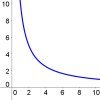### Graphs of Changing Areas

##### Age 16 to 18 Challenge Level:

Use graphs to gain insights into an area and perimeter problem, or use your knowledge of area and perimeter to gain insights into the graphs...### Interpolating Polynomials

##### Age 16 to 18 Challenge Level:

Given a set of points (x,y) with distinct x values, find a polynomial that goes through all of them, then prove some results about the existence and uniqueness of these polynomials.### Parabella

##### Age 16 to 18 Challenge Level:

This is a beautiful result involving a parabola and parallels.### Intersections

##### Age 14 to 18 Challenge Level:

Change one equation in this pair of simultaneous equations very slightly and there is a big change in the solution. Why?##### Age 14 to 16 Challenge Level:

Four vehicles travelled on a road. What can you deduce from the times that they met?### Matchless

##### Age 14 to 16 Challenge Level:

There is a particular value of x, and a value of y to go with it, which make all five expressions equal in value, can you find that x, y pair ?### Climbing

##### Age 16 to 18 Challenge Level:

Sketch the graphs of y = sin x and y = tan x and some straight lines. Prove some inequalities.### Cubic Spin

##### Age 16 to 18 Challenge Level:

Prove that the graph of f(x) = x^3 - 6x^2 +9x +1 has rotational symmetry. Do graphs of all cubics have rotational symmetry?##### Age 14 to 16 Challenge Level:

Four vehicles travel along a road one afternoon. Can you make sense of the graphs showing their motion?### Small Steps

##### Age 16 to 18 Challenge Level:

Two problems about infinite processes where smaller and smaller steps are taken and you have to discover what happens in the limit.### Lap Times

##### Age 14 to 16 Challenge Level:

Can you find the lap times of the two cyclists travelling at constant speeds?### Exploring Cubic Functions

##### Age 14 to 18 Challenge Level:

Quadratic graphs are very familiar, but what patterns can you explore with cubics?### Parabolas Again

##### Age 14 to 18 Challenge Level:

Here is a pattern composed of the graphs of 14 parabolas. Can you find their equations?### Equation Matcher

##### Age 16 to 18 Challenge Level:

Can you match these equations to these graphs?### More Parabolic Patterns

##### Age 14 to 18 Challenge Level:

The illustration shows the graphs of twelve functions. Three of them have equations y=x^2, x=y^2 and x=-y^2+2. Find the equations of all the other graphs.### Parabolic Patterns

##### Age 14 to 18 Challenge Level:

The illustration shows the graphs of fifteen functions. Two of them have equations y=x^2 and y=-(x-4)^2. Find the equations of all the other graphs.### Motion Sensor

##### Age 14 to 16 Challenge Level:

Looking at the graph - when was the person moving fastest? Slowest?### Bio Graphs

##### Age 14 to 16 Challenge Level:

What biological growth processes can you fit to these graphs?### Maths Filler 2

##### Age 14 to 16 Challenge Level:

Can you draw the height-time chart as this complicated vessel fills with water?### Immersion

##### Age 14 to 16 Challenge Level:

Various solids are lowered into a beaker of water. How does the water level rise in each case?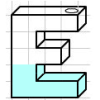### Mathsjam Jars

##### Age 14 to 16 Challenge Level:

Imagine different shaped vessels being filled. Can you work out what the graphs of the water level should look like?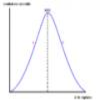### Graphic Biology

##### Age 16 to 18 Challenge Level:

Several graphs of the sort occurring commonly in biology are given. How many processes can you map to each graph?### Which Is Bigger?

##### Age 14 to 16 Challenge Level:

Which is bigger, n+10 or 2n+3? Can you find a good method of answering similar questions?### What's That Graph?

##### Age 14 to 16 Challenge Level:

Can you work out which processes are represented by the graphs?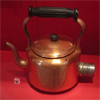### Electric Kettle

##### Age 14 to 16 Challenge Level:

Explore the relationship between resistance and temperature### Whose Line Graph Is it Anyway?

##### Age 16 to 18 Challenge Level:

Which line graph, equations and physical processes go together?### Exponential Trend

##### Age 16 to 18 Challenge Level:

Find all the turning points of y=x^{1/x} for x>0 and decide whether each is a maximum or minimum. Give a sketch of the graph.### Curve Match

##### Age 16 to 18 Challenge Level:

Which curve is which, and how would you plan a route to pass between them?### Curve Fitter

##### Age 16 to 18 Challenge Level:

Can you fit a cubic equation to this graph?### Real-life Equations

##### Age 16 to 18 Challenge Level:

Here are several equations from real life. Can you work out which measurements are possible from each equation?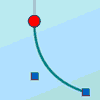### Graphical Interpretation

##### Age 14 to 16 Challenge Level:

This set of resources for teachers offers interactive environments to support work on graphical interpretation at Key Stage 4.### Golden Construction

##### Age 16 to 18 Challenge Level:

Draw a square and an arc of a circle and construct the Golden rectangle. Find the value of the Golden Ratio.### Bus Stop

##### Age 14 to 16 Challenge Level:

Two buses leave at the same time from two towns Shipton and Veston on the same long road, travelling towards each other. At each mile along the road are milestones. The buses' speeds are constant. . . .### Which Is Cheaper?

##### Age 14 to 16 Challenge Level:

When I park my car in Mathstown, there are two car parks to choose from. Can you help me to decide which one to use?### Ellipses

##### Age 14 to 18 Challenge Level:

Here is a pattern for you to experiment with using graph drawing software. Find the equations of the graphs in the pattern.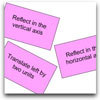### Surprising Transformations

##### Age 14 to 16 Challenge Level:

I took the graph y=4x+7 and performed four transformations. Can you find the order in which I could have carried out the transformations?### Diamond Collector

##### Age 11 to 16 Challenge Level:

Collect as many diamonds as you can by drawing three straight lines.### Guess the Function

##### Age 16 to 18 Challenge Level:

This task depends on learners sharing reasoning, listening to opinions, reflecting and pulling ideas together.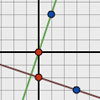### Perpendicular Lines

##### Age 14 to 16 Challenge Level:

Position the lines so that they are perpendicular to each other. What can you say about the equations of perpendicular lines?